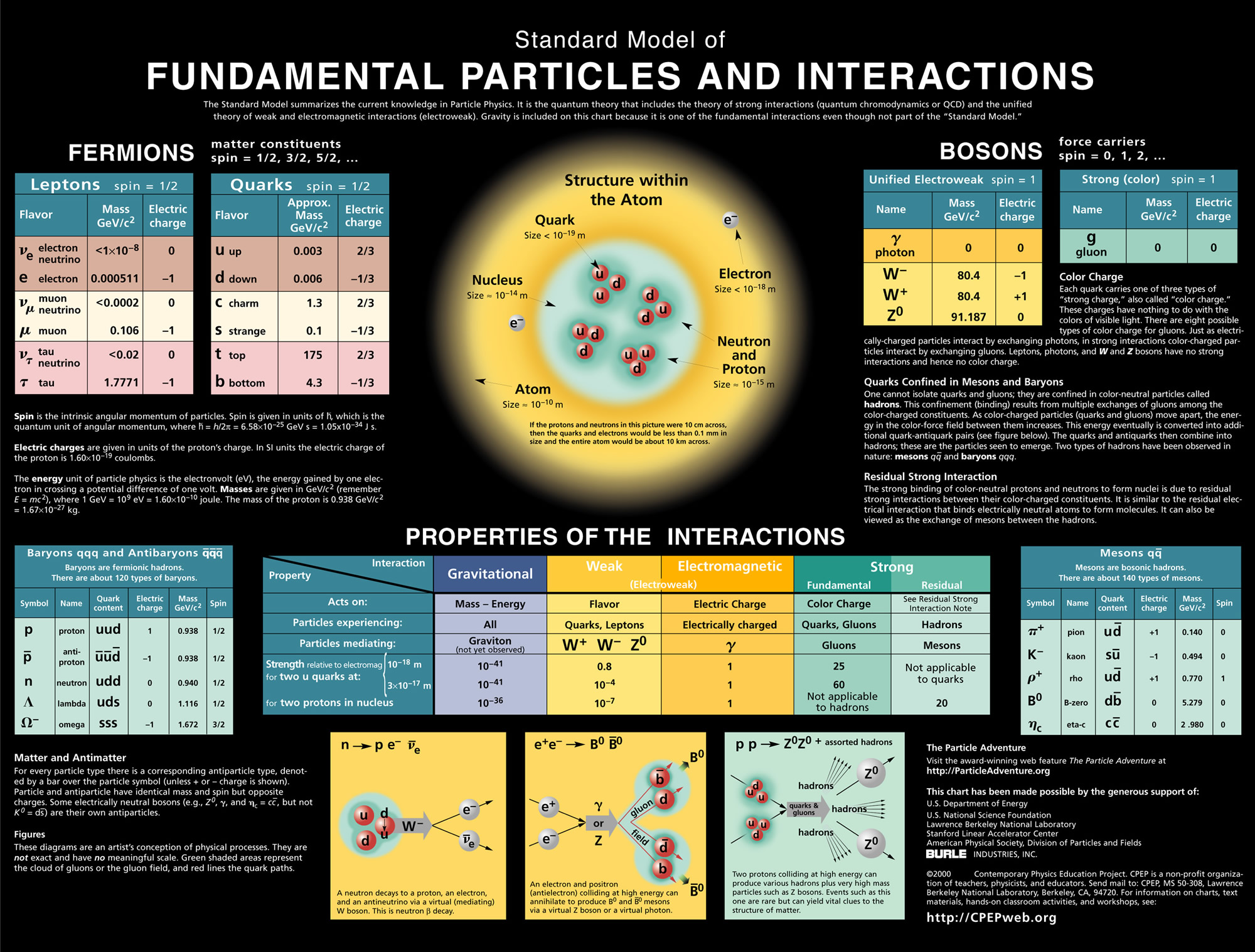# Topic 7: Atomic, nuclear and particle physics

See the guide for this topic.

## 7.1 – Discrete energy and radioactivity

• ### Discrete energy and discrete energy levels

• The electrons of an atom can occupy certain discrete atomic energy levels.
• As an electron makes a jump from one energy level to another, energy is absorbed or released in the form of a photon. The amount of energy absorbed or released is equal to the difference between the discrete atomic energy levels and is also quantized.
• The energy of a photon is dependent on its frequency. Therefore, only photons with frequencies which correspond to the differences between the atomic energy levels can be absorbed or released by an atom. These frequencies appear as spectral lines in the emission and absorption spectra.• The existence of discrete energy levels called atomic energy levels can be supported by the emission spectra and the absorption spectra of atoms.• ### Transitions between energy levels

When the electrons within an atom jump from one atomic energy level to a lower energy level, energy is released in the form of light. Likewise, light is absorbed when the electrons within an atom jump from one atomic energy level to a higher energy level.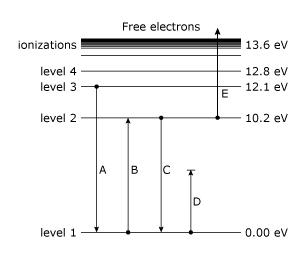The amount of energy absorbed or released can be calculated by the difference in energy (eV) between the two energy levels.

• Radioactive decay refers to the spontaneous random process by which particles or electromagnetic radiation is emitted from an unstable nucleus. The product nucleus from a radioactive decay is called a daughter nucleus.
• The daughter nucleus is energetically unstable.
• The activity of radioactive decay can be shown by half-lives.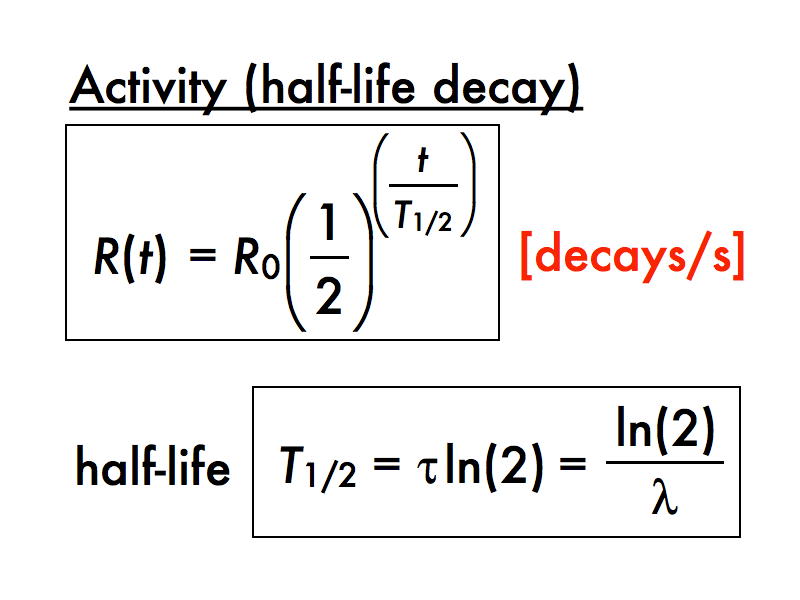• The radioactive half-life of a substance is the time it takes for half of its radioactive nuclei to decay.
• If we plot the amount of radioactive nuclei which have not yet decayed with time, the resulting curve is called the decay curve and can be shown as the following.• ### Fundamental forces and their properties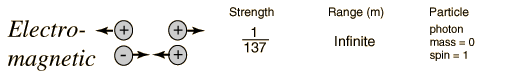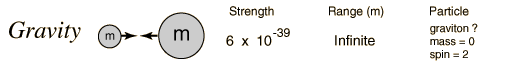• ### Alpha particles, beta particles and gamma rays

Alpha particles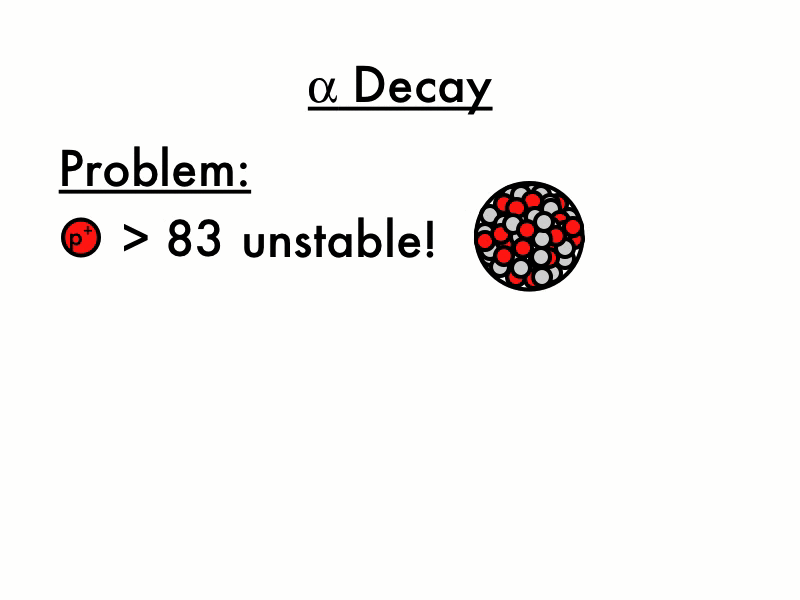An alpha particle is a helium nucleus.

• It has a relative charge of +2.
• Its penetration power is the lowest among the three types of particles and can be blocked by a piece of paper or a few cm of air.
• Its ionizing power is the highest among the three types of particles.

Beta particles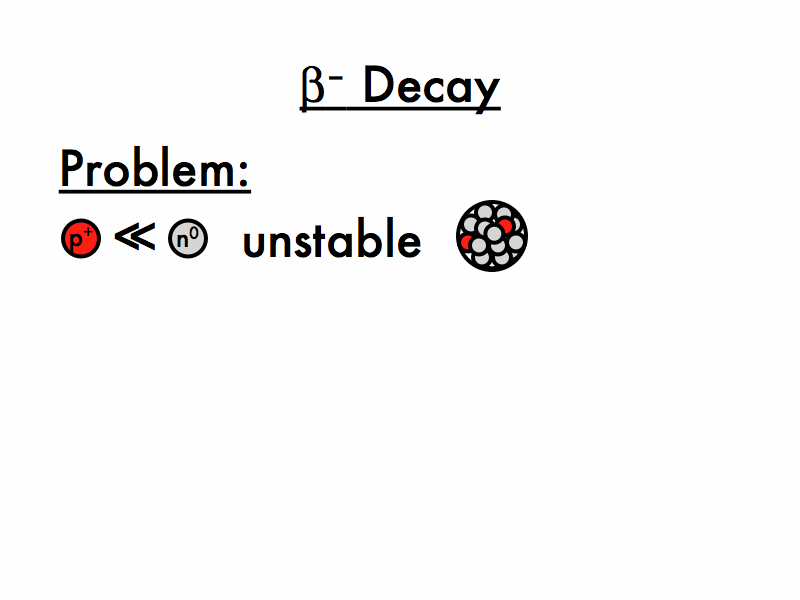A beta particle is an electron or a positron.

• It has a relative charge of -1 or +1.
• Its penetration power is in the middle among the three types of particles and can be blocked by a thin sheet of aluminum.
• Its ionizing power is in the middle among the three types of particles.

Gamma rays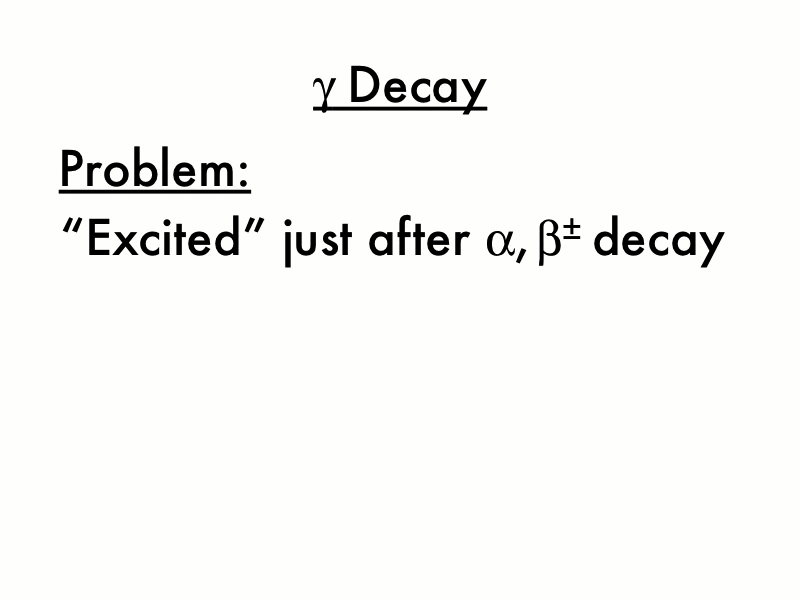• Gamma rays are photons.
• It does not have a charge.
• Its penetration power is the highest among the three types of particles and can be blocked by several cm of lead.
• Its ionizing power is in the lowest among the three types of particles.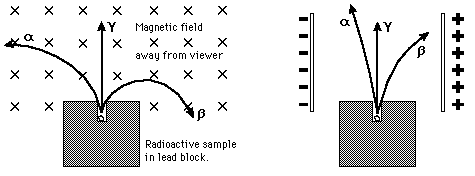• ### Absorption characteristics of decay particles

 Short-term effects Long-term effects    Radiation burn    Nausea and vomiting    Diarrhea    Headache    Cancer    Genetic mutations
• ### Isotopes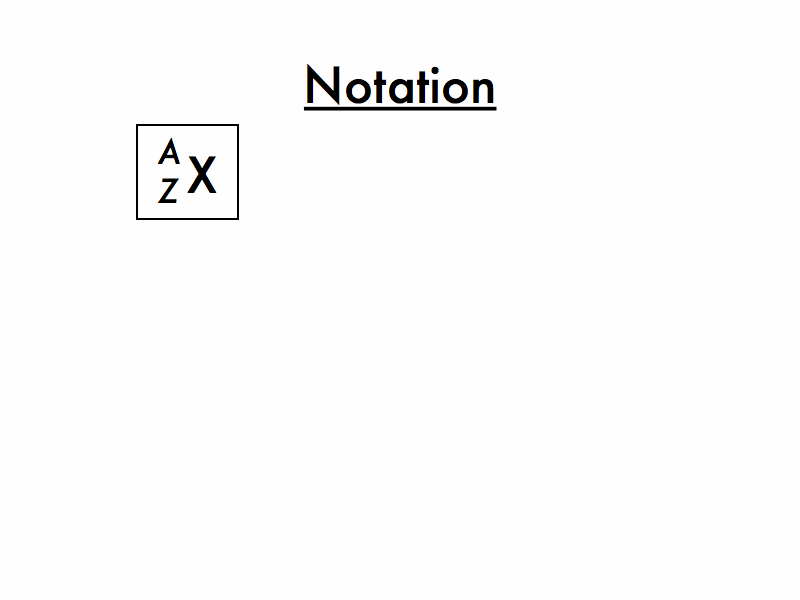Different isotopes of a given element have the same atomic number (atomic number defines the type of element) but different mass numbers because they have different numbers of neutrons.

Background radiation comes from natural sources and artificial sources.

• Natural sources: cosmic rays from space, radioactive rocks and soil, living organisms that have consumed radioactive substances in the food chain
• Artificial sources: radioactive waste from nuclear power plants, radioactive fallout from nuclear weapons, medical x-rays

Average composition and exposure of background radiation## 7.2 – Nuclear reactions

• ### The unified atomic mass unit

The unified atomic mass unit (μ) is commonly used in nuclear physics. It is defined as one twelfth of the mass of a carbon-12 atom.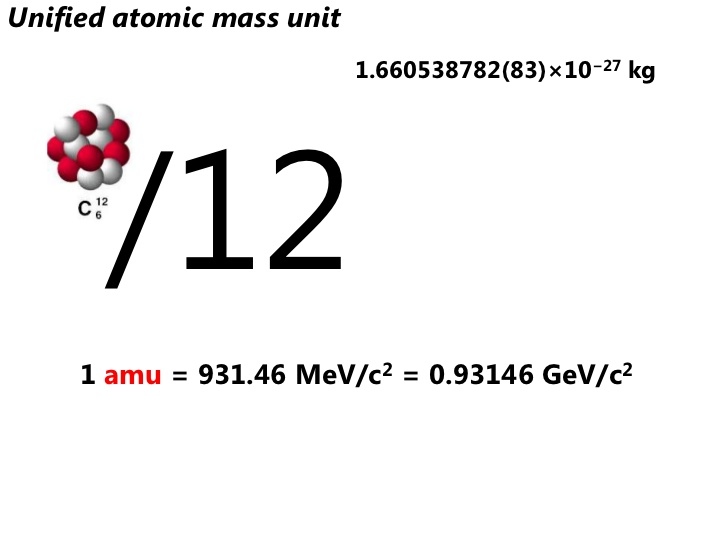• ### Mass defect and nuclear binding energy

Mass defect

• The difference between the mass of an atom and the sum of mass of its constituent parts is called its mass defect.
• Mass defect can be explained by Einstein’s mass-energy equivalence: As energy required to break apart a nucleus, the sum of energy contained in the constituent nucleons is higher than that of the combined nucleus. Energy is related to mass.

Nuclear binding energy

• The nuclear binding energy of a nucleus is the amount of work required to separate the nucleons inside the nucleus.
• Binding energy per nucleon = binding energy of nucleus / number of nucleons in nucleus.where E is energy in J, m is mass in kg, and c is the speed of light in m/s

### FYI

The nuclear binding energy curve• ### Nuclear fission and nuclear fusion

Nuclear fission

• Nuclear fission is the splitting of a heavy nucleus into two lighter nuclei.
• A great amount of energy is released in nuclear fission due to the greater binding energy of the daughter nuclei.
• Nuclear fission is used in nuclear power plants.

Nuclear fusion

• Nuclear fusion is the joining of two light nuclei to form a heavy nucleus.
• A great amount of energy is released in nuclear fusion due to the greater binding energy of the daughter nucleus.
• Nuclear fusion yields more energy than nuclear fission.
• Nuclear fusion is the main source of the sun’s energy.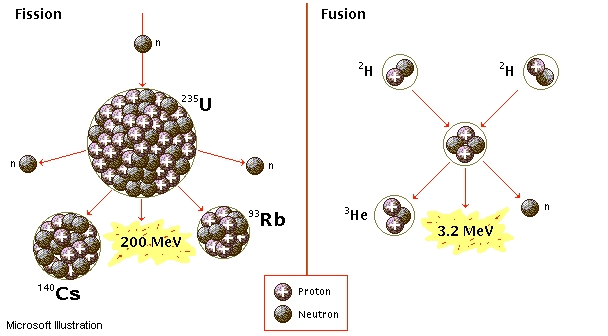## 7.3 – The structure of matter

• ### Quarks, leptons and their antiparticles

• Quarks and leptons are the elementary particles (building blocks) of matter.
• Each particle also has an antimatter counterpart.
• When matter collides with its corresponding antimatter, the particles annihilate and release energy by mass-energy equivalence.• ### Hadrons, baryons and mesons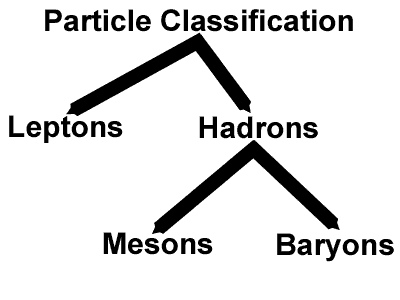• As stated in the previous section, the elementary particles of matter comprises of quarks and leptons.
• Hadrons are made up of quarks and are identified in the same classification level as leptons.
• Unlike leptons, which do not experience the strong nuclear force, hadrons experience all four fundamental forces.
• Hadrons are generally larger than leptons.
• Hadrons are sub-divided into baryons and mesons.
• Baryons and mesons are made up of different types of quarks and antiquarks.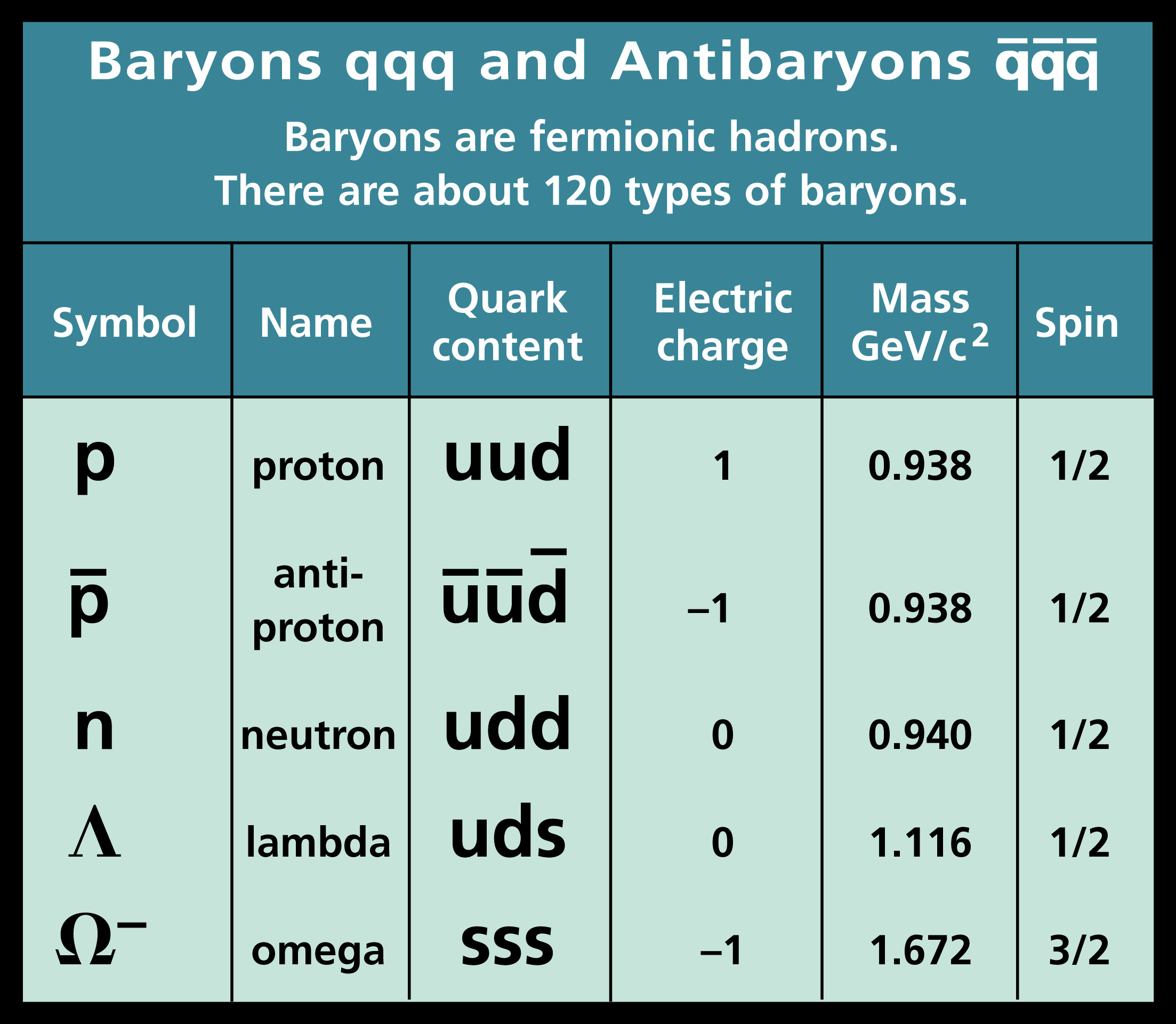where u represents up quarks, d represents down quarks, c represents charm quarks, s represents strange quarks, (t represents top quarks), b represents bottom quarks, and the line above the representative letter of the quarks indicate its corresponding antiquarks.

• The conservation laws of charge, baryon number, lepton number and strangeness
• When writing equations, we already know that the charge of the reactants and the products must be identical due to the conservation law of charge.
• In particle physics, other than the conservation of charge, the baryon number, lepton number, and strangeness must also be conserved.
• Baryons have a baryon number of +1, antibaryons have a baryon number of -1, leptons have a lepton number of +1, and antileptons have a lepton number of -1.
• The conservation of strangeness (strange quark) only occurs within interactions of the strong nuclear force while the conservation of the other three properties apply to all interactions.
• ### The nature and range of the strong nuclear force, weak nuclear force and electromagnetic force

See previous section in 7.2 (Fundamental forces and their properties).

• ### Exchange particles

Exchange particles of the four fundamental forces are gluons, photons, W+ bosons, W- bosons, Z0 bosons, and gravitons.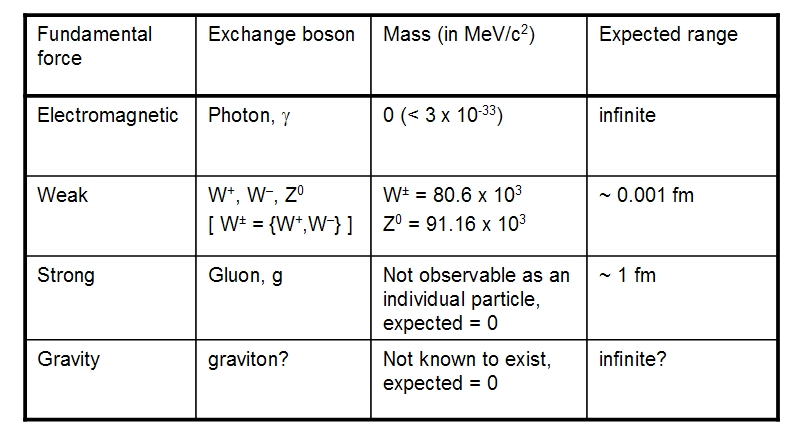See previous section in 7.2 (Fundamental forces and their properties).

• ### Feynman diagrams

• Feynman diagrams, introduced by physicist Richard Feynman, can be used to express the behavior of subatomic particles over time.
• Feynman diagrams are read from the left to the right where the x-axis shows time and the y-axis shows roughly the space direction of the subatomic particle interactions. Some Feynman diagrams, such as the examples given below, switch the x-axis and the y-axis where the progression of the interactions with time is read upwards.Some examples of Feynman diagrams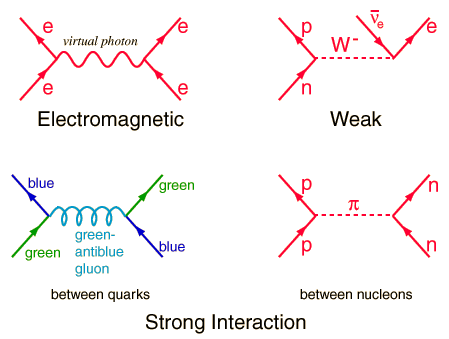For a comprehensive guide on how to draw Feynman diagrams, visit http://www.quantumdiaries.org/2010/02/14/lets-draw-feynman-diagams/.

• ### Confinement

Quarks and gluons (massless subatomic particles that transmit the force binding quarks together in a hadron) are color-charged particles. Similar to electrically-charged particles which interact by exchanging photons in electromagnetic interactions, color-charged particles exchange gluons in strong force interactions. Note that color charge has nothing to do with visible colors. It is just an expression.

When two quarks are close to each other, they exchange gluons and create a strong color force field that binds quarks together. The force field gets stronger as the quarks get further apart. Quarks constantly change their color charges as they exchange gluons with other quarks. There are 3 color charges and 3 corresponding anti-color charges.Just as mixing red, blue, and green visible colors yield white, mixing red, blue, and green color charges yield color neutral.

Color confinement is a phenomenon that color-charged particles cannot be isolated singularly and therefore cannot be directly observed. The color-charged quarks are said to be confined in groups (hadrons) with other quarks which composite to color neutral and cannot be distinguished separately. This is because the color force increases as the color-charged quarks are pulled apart.

TL;DR: Color confinement or quark confinement is the phenomenon when isolated quarks and gluons cannot be observed.

• ### The Higgs boson

In addition to the three generations of leptons and quarks (see previous section (Quarks, leptons and their antiparticles)), there are four classes of bosons and an additional highly massive boson called the Higgs boson. This particle was proposed in 1964 to explain the process which particles can acquire mass and was identified with the Large Hadron Collider (LHC).### FYI

The Large Hadron Collider (LHC) is the world’s largest and most powerful particle collider, the largest and most complex experimental facility ever built, and the largest single machine in the world. It was built by CERN in collaboration with over 10000 scientists and engineers from over 100 countries along with hundreds of universities and laboratories.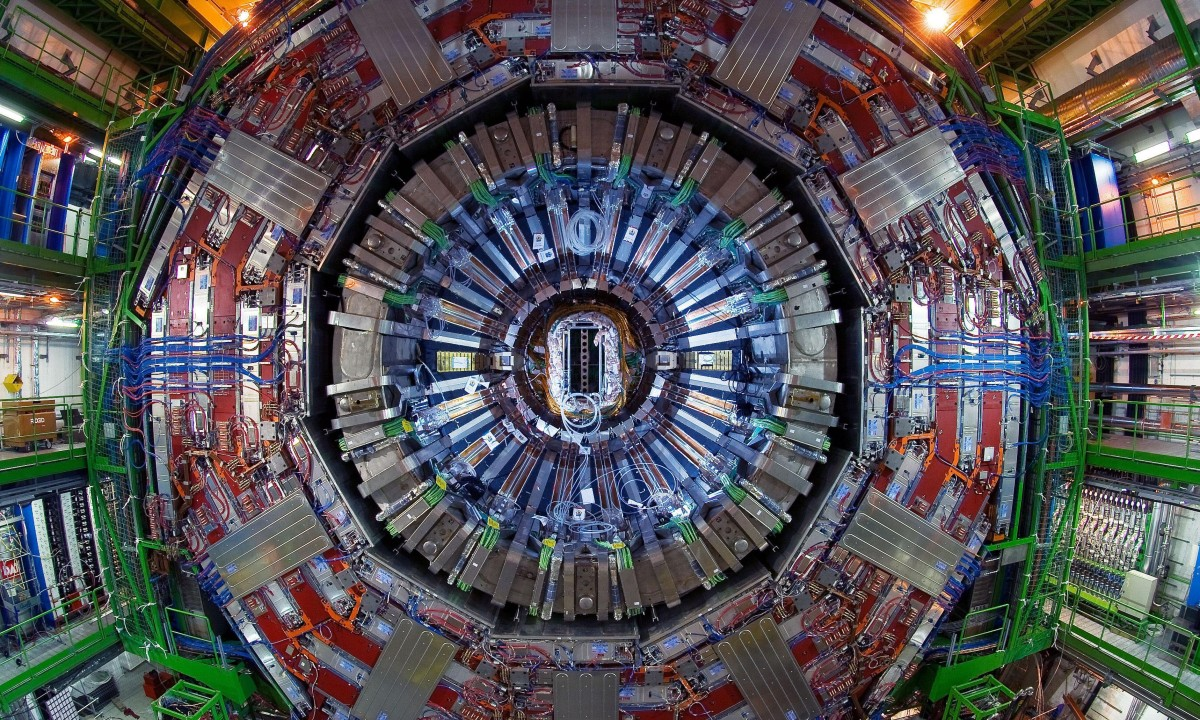Our changing views of the atom model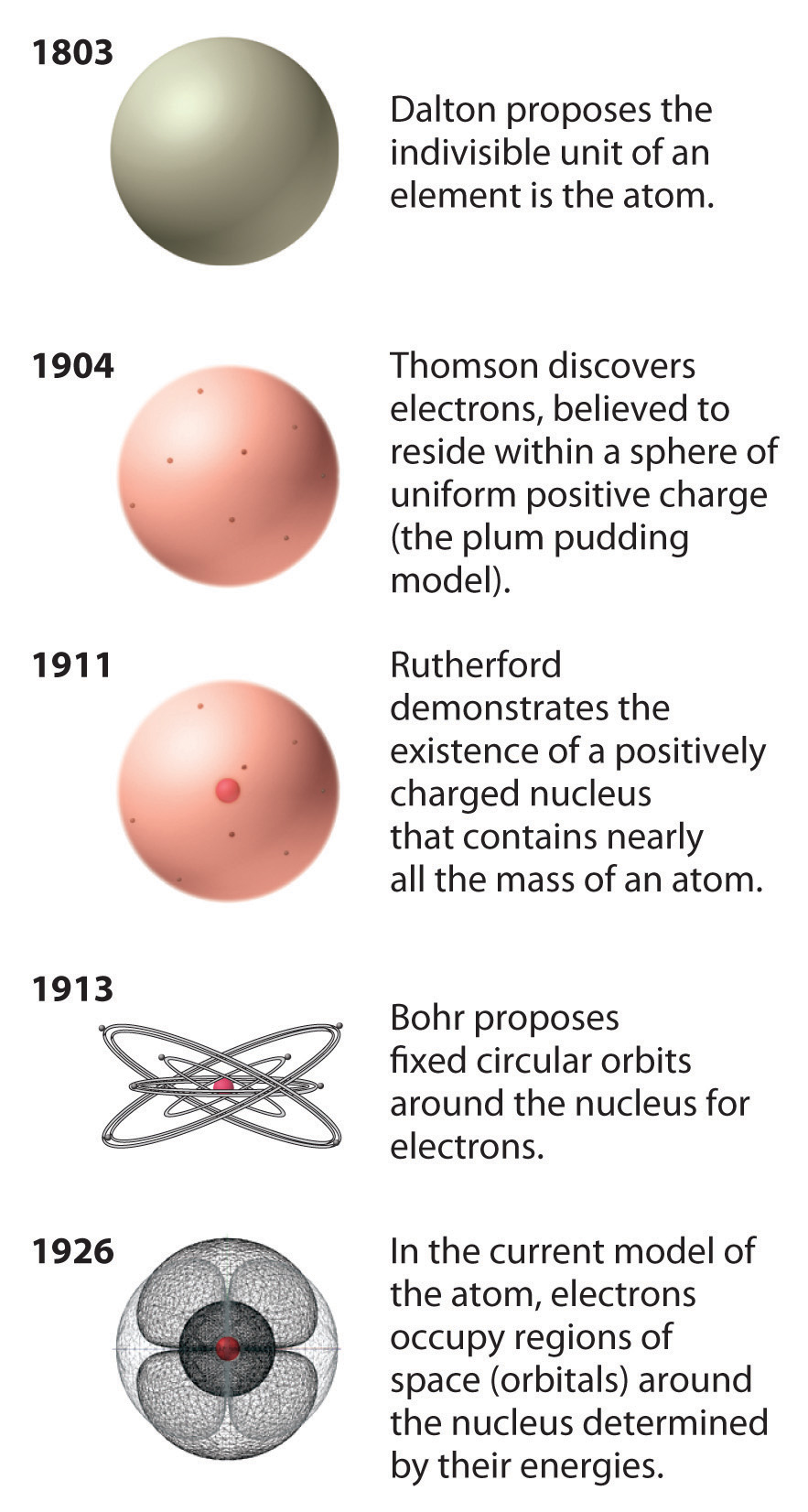Summary of fundamental particles and interactions